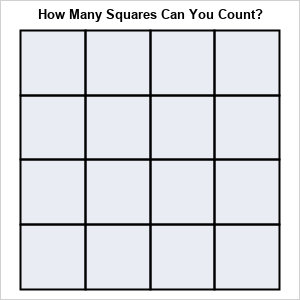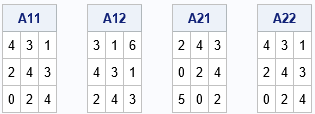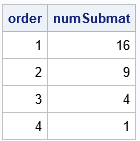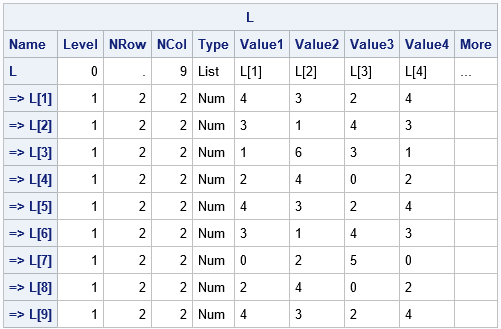Have you ever seen the "brain teaser" for children that shows a 4 x 4 grid and asks "how many squares of any size are in this grid?" To solve this problem, the reader must recognize that there are sixteen 1 x 1 squares, nine 2 x 2 squares, four 3 x 3 squares, and one 4 x 4 square. Hence there are a total of (16+9+4+1) = 30 squares of any size.

Recently a SAS statistical programmer asked a similar question about submatrices of a matrix. Specifically, the programmer wanted to form square submatrices of consecutive rows and columns. This article shows a few tricks in the SAS/IML language that enable you to generate and compute with all k x k submatrices of a matrix, where the submatrices are formed by using consecutive rows and columns of the original matrix. Although it is possible to consider more general submatrices, in this article, a "submatrix" always refers to one that is formed by using consecutive rows and columns.

### Use subscripts to form submatrices

I've previously written about several ways to extract submatrices from a matrix. Recall the "colon operator" (formally called the index creation operator) generates a vector of consecutive integers. You can use the vectors in the row and column position of the subscripts to extract a submatrix. For example, the following SAS/IML statements define a 4 x 4 matrix and extract the four 3 x 3 submatrices:

```A = {4 3 1 6, 2 4 3 1, 0 2 4 3, 5 0 2 4}; /* extract the four 3 x 3 submatrices */ A11 = A[1:3, 1:3]; A12 = A[1:3, 2:4]; A21 = A[2:4, 1:3]; A22 = A[2:4, 2:4];```

Manually specifying the row and column numbers is tedious. Let's write a SAS/IML function that will return a k x k submatrix of A by starting at the (i,j)th position of A. Call k the order of the submatrix.

```/* Given A, return the square submatrix of order k starting at index (i,j) */ start submat(A, i, j, k); return A[ i:(i+k-1), j:(j+k-1) ]; finish;   /* example : 3 x 3 submatrices of A */ A11 = submat(A, 1, 1, 3); A12 = submat(A, 1, 2, 3); A21 = submat(A, 2, 1, 3); A22 = submat(A, 2, 2, 3);   /* https://blogs.sas.com/content/iml/2015/12/02/matrices-graphs-gridded-layout.html */ ods layout gridded columns=4 advance=table; print A11, A12, A21, A22; ods layout end;```### The number of submatrices

If A is an n x m matrix, we can ask how many submatrices of order k are in A. Recall that we are only considering submatrices formed by consecutive rows and columns. There are (n – k + 1) sequences of consecutive rows of length k, such as 1:k, 2:(k+1), and so forth. Similarly, there are (m – k + 1) sequences of consecutive columns of length k. So the following SAS/IML function counts the number of submatrices of order k. You can call the function for different k=1, 2, 3, and 4 in order to solve the "Counting Squares" brain teaser:

```/* count all submatrices of order k for the matrix A */ start CountAllSubmat(A, k); numRows = nrow(A)-k+1; numCols = ncol(A)-k+1; return numRows * numCols; finish;   n = nrow(A); numSubmat = j(n, 1, .); do k = 1 to n; numSubmat[k] = CountAllSubmat(A, k); end; order = T(1:n); print order numSubmat;```If you add up the number of squares each size, you get a total of 30 squares.

### Iterate over submatrices of a specified order

You can iterate over all submatrices of a specified order. You can perform a matrix computation on each submatrix (for example, compute its determinant), or you can pack it into a list for future processing. The following SAS/IML function iterates over submatrices and puts each submatrix into a list. The STRUCT subroutine from the ListUtil package can be used to indicate the contents of the list in a concise form.

```start GetListSubmat(A, k); numSub = CountAllSubmat(A, k); L = ListCreate( numSub ); /* create list with numSub items */ cnt = 1; do i = 1 to nrow(A)-k+1; /* for each row */ do j = 1 to ncol(A)-k+1; /* and for each column */ B = submat(A, i, j, k); /* compute submatrix of order k from (i,j)th cell */ call ListSetItem(L, cnt, B); /* put in list (or compute with B here) */ cnt = cnt + 1; end; end; return L; finish;   /* Example: get all matrices of order k=2 */ L = GetListSubmat(A, 2);   package load ListUtil; call Struct(L);```The output from the STRUCT call shwos that there are nine 2 x 2 matrices. The Value1–Valuie4 columns show the values (in row-major order). For example, the first 2 x 2 submatrix (in the upper left corner of A) is {4 3, 2 4}. The second submatrix (beginning with A[1,2]) is {3 1, 4 3}.

It is straightforward to modify the function to compute the determinant or some other matrix computation.

### Summary

This article shows some tips and techniques for dealing with submatrices of a matrix. The submatrices in this article are formed by using consecutive rows and columns. Thus, they are similar to the "Counting Squares" brain teaser, which requires that you consider sub-squares of order 1, 2, 3, and so forth.

The post Submatrices of matrices appeared first on The DO Loop.